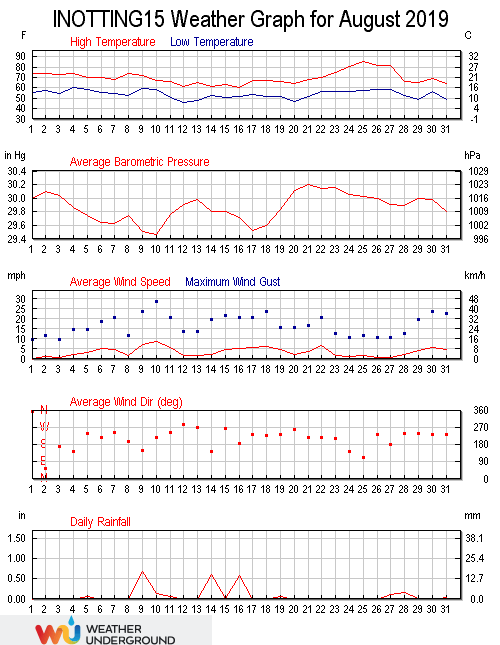August 2019 was warmer than average (16.7°C versus an average of 15.5), wetter than average (63.00 mm versus an average total of 49.91) and windier than average (a wind run of 2660 miles versus an average of 2272).

The highest temperature in the month was 30.0°C recorded at 17:54 on the 25th, the second highest temperature of 2019, beaten only by the 34.1°C recorded on July 25th. This is only the sixth time that a temperature of 30.0°C has been recorded here. The minimum temperature was 8.1°C, recorded at 06:04 on the 12th. The average temperature in the month was 16.7°C, 1.1°C above the August mean and a high for August. The average daily high temperature was 21.3°C, 0.8°C above the mean, with the average daily low being 12.4°C, 0.8°C above the mean, both August highs. The warmest day was the 25th with an average temperature of 22.3°C, with the coolest day being the 12th with an average temperature of 12.3°C.

The average wind chill in the month was 16.6°C, and the average dew point was 13.2°C. The high dew point was 19.9°C recorded at 12.29 on the 27th. The low dew point was 7.0°C at 16:04 on the 12th. There were 207.2 hours of sunshine in the month, with the sunniest day being the 24th with 10.3 hours. The maximum heat index was 30.4°C recorded at 18:08 on the 25th.

Rainfall in the month was 63.0 millimetres, 11.9 millimetres above the August average. Rainfall was recorded on 12 days, with the wettest day being the 9th when 17.2 millimetres of rain fell. The year to date total is 423 millimetres, 118% above the average for the end of August. The highest rain rate was 67.8 millimetres per hour, recorded at 22:28 on the 27th.

The highest barometric pressure of 1024.8 millibars was at 10:31 on the 21st. The lowest pressure was 991.2 millibars at 03:06 on the 10th. The average pressure in the month was 1011.3 millibars. The highest humidity was 96% at 09:00 on the 29th, and the monthly average humidity was 81%. The lowest humidity was 40% at 15:00 on the 25th.

Winds were as expected in the month, with the highest wind gust speed being 29 mph recorded at 10:12 on the 10th, 0.6 mph above the month average. The maximum wind speed was 16 mph at 16:16 on the 10th, 0.8 mph below the mean. The wind run was 2660.2 miles, well above the August average of 2490.1 miles. The average gust speed in the month was 6.0 mph, 0.5 mph above the mean. The average wind speed was 4.0 mph, 0.6 mph above the mean. The predominant direction was south south-westerly (208°).

The maximum high solar radiation recorded at 12:45 on the 9th was 1090 w/m². The average solar radiation for the month was 175 w/m². The highest U.V. Index was 4.9 recorded at 12:44 on the 9th, well below the August average of 6.0.This site uses Akismet to reduce spam. Learn how your comment data is processed.